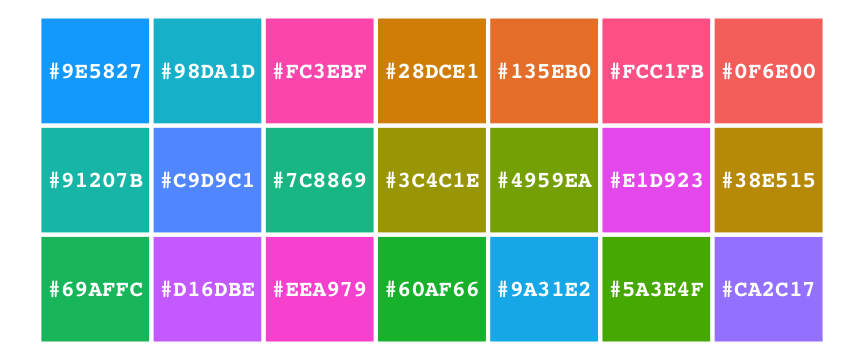# Generate a large color palette with Colorgorical

2018-07-23Sometimes we need a lot of colors to represent all the categories in our data. We can use the httr and jsonlite packages to retrieve a list of colors from the Colorgorical website by Connor Gramazio.

Let’s use the web API to retrieve a color palette:

# install.packages(c("httr", "jsonlite"))

colorgorical <- function(n = 10) {
# Create a JSON data string that we'll send to the server.
post_body <- jsonlite::toJSON(
auto_unbox = TRUE,
list(
'paletteSize' = n,
'weights' = list(
'ciede2000' = 0,
'nameDifference' = 0,
'nameUniqueness' = 0,
'pairPreference' = 0
),
'hueFilters' = list(),
'lightnessRange' = c("25", "85"),
'startPalette' = list()
)
)
# Send a POST request to the server with our data.
retval <- httr::POST(
url = 'http://vrl.cs.brown.edu/color/makePalette',
body = post_body
)
# Get the response from the server.
retval <- httr::content(retval)
# Convert LAB values to hexadecimal strings.
lab2hex <- function(Lab) rgb(convertColor(Lab, from = "Lab", to = "sRGB"))
return(sapply(retval\$palette, function(x) {
lab2hex(unlist(x[1:3]))
}))
}

pal <- colorgorical(21)

d <- data.frame(x = rep(1:7, 3), y = rep(1:3, each = 7), color = pal)

library(ggplot2)

ggplot(d, aes(x, y, fill = color)) +
geom_tile(color = "white", size = 2) +
geom_text(
mapping = aes(label = color),
color = "white", size = 8, fontface = "bold", family = "Courier"
) +
theme_void() +
theme(legend.position = "none")You should also try http://tools.medialab.sciences-po.fr/iwanthue/